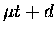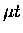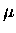Next: Time-of-flight analysis Up: Fusion yield analysis Previous: US yield

### MOD yield

In the MOD yield analysis, before comparing with the MC yield, we used a simple attenuation model to analyze our data, which gave us crude yet useful insight into the physics involved.

In the one dimensional approximation, the yield Y of particles after going through a medium of thickness d can be expressed as:

 (113)

where A0 is the number of the original particle, d medium thickness, and the L att, the attenuation interaction length. The attenuation interaction is defined here as a process which absorbs or deflects the particle.

Fitting our data using Eq. 8.7 gave us a rather good fit with (confidence level 19%, dof=2), which is somewhat surprising, considering the degree of approximation introduced. Nonetheless, with some caution in its interpretation, we can extract a phenomenological parameter, the attenuation interaction length incollisions gcm-2, where the first error is given by the fit, and the second error is due to the uncertainty in the layer thickness (the latter was obtained from different fits in which the thickness was varied). The error in the experimental yield used in the fit included only the relative uncertainties, since normalization errors such as muon stopping fraction and Si solid angle cancel in the thickness dependence. The value of L eff int can be converted to the effective attenuation interaction cross section cm-2, using with n the number density. This can be compared to thetotal elastic scattering cross section  - cm-2 atlab energies of 1-20 eV, near whichemission is distributed. The difference in the two cross sections can be in part understood in that it takes more than one collision to attenuate the, especially given the scattering angular distribution, which we will shortly come to.

We now turn to the comparisons with the SMC simulations. Encouraged by its success in fitting the experimental data, we apply the attenuation model also to characterize the MC results. Figure 8.18 illustrates our Monte Carlo analysis. Plotted with error bars are experimental data, while crosses indicate the yield from the MC. The lines are fits to the MC yield from which we extract the effective attenuation lengths as above. Shown in the solid line (MC(a)) is a fit to the MC results with the nominal input, with dotted lines indicating the variations in the slope due to the fit uncertainty and the thickness errors, where we obtainedgcm-2. This is consistent at the 10% level with the experimental value extracted above. Indeed, the same conclusion can be made from a direct comparison of the Monte Carlo and the data without the use of the intermediate approximation of the attenuation model.

On the other hand, given in the dot-dashed line is a fit to the MC assuming an isotropic angular distribution inelastic scattering with the total cross section kept the same (MC(b)), where we obtainedgcm-2. The Monte Carlo with an isotropic angular distribution is in disagreement with our data. In Table 8.15 we present the summary of our attenuation analysis. We shall defer the discussion of the implications of these measurements to Chapter 9, but for now it suffices to state that a reasonable agreement of our Leffint with the MC gives us some confidence in our model ofmoderation in a deuterium layer.Next: Time-of-flight analysis Up: Fusion yield analysis Previous: US yield